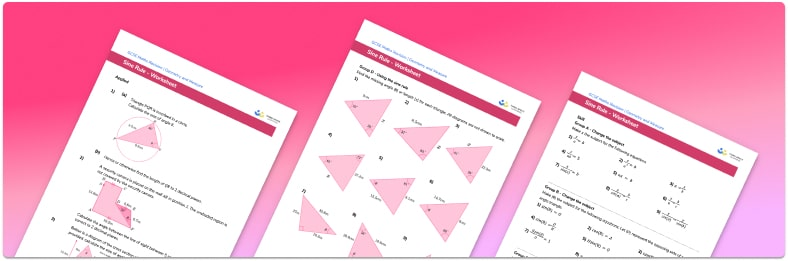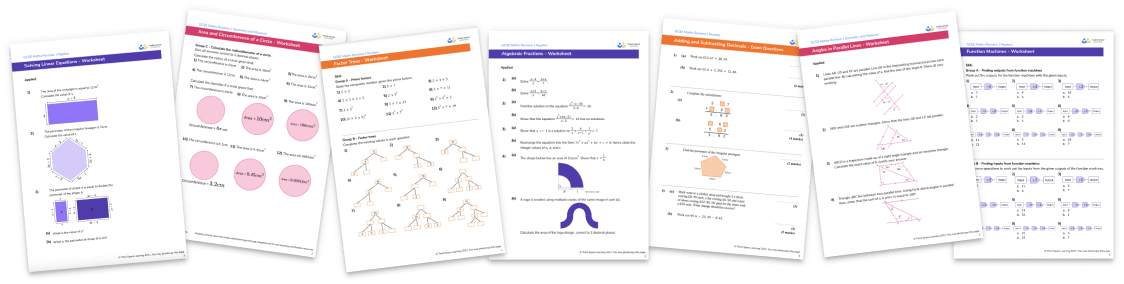# Sine Rule Worksheet• Section 1 of the sine rule worksheet contains 36 skills-based sine rule questions, in 4 groups to support differentiation
• Section 2 contains 3 applied sine rule questions with a mix of worded problems and deeper problem solving questions
• Answers and a mark scheme for all sine rule questions are provided and include higher maths questions based on past papers, exam questions and assessments
• Questions follow variation theory with plenty of opportunities for students to work independently at their own level
• All questions created by fully qualified expert secondary maths teachers
• Suitable for GCSE maths revision for AQA, OCR and Edexcel exam boards

• This field is for validation purposes and should be left unchanged.

You can unsubscribe at any time (each email we send will contain an easy way to unsubscribe). To find out more about how we use your data, see our privacy policy.

### Sine rule at a glance

The sine rule is a relationship between the sides of a triangle and the angles opposite them. The sine rule states that ‘’a/sin(A) = b/sin(B) = c/sin(C)’’ where a, b and c are the lengths of the sides and A, B and C are the angles opposite them.

The sine rule can be used to calculate missing sides and missing angles in non right-angled triangles. The sine rule is used when one angle – side pair is known along with one other angle or side. The opposite side or angle can then be worked out. Although the sine rule states three relationships between the sides and their opposite angles, we only need to use two of them at a time.

When finding a missing side length, we can substitute the information we know about other angles and sides into the sine rule and then solve the equation to find the side length. When working out the size of the angle, solving the equation will involve using the inverse trigonometric function.

When working with the sine rule, it is important not to round your answers too early in your calculation and to pay attention to the required number of significant figures or decimal places for your answer.

Looking forward, students can then progress to additional geometry worksheets, for example, a 3D shapes worksheet orFor more teaching and learning support on Geometry our GCSE maths lessons provide step by step support for all GCSE maths concepts.

## Do you have KS4 students who need more focused attention to succeed at GCSE?There will be students in your class who require individual attention to help them succeed in their maths GCSEs. In a class of 30, it’s not always easy to provide.

Help your students feel confident with exam-style questions and the strategies they’ll need to answer them correctly with our dedicated GCSE maths revision programme.

Lessons are selected to provide support where each student needs it most, and specially-trained GCSE maths tutors adapt the pitch and pace of each lesson. This ensures a personalised revision programme that raises grades and boosts confidence.

Find out more